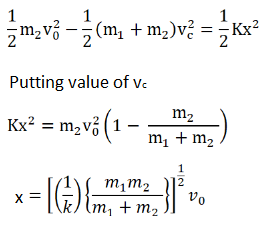# HC Verma Solutions Class 11 Chapter 9 Centre of Mass, Linear Momentum, Collision

HC Verma Class 11 Solutions Chapter 9 Center of Mass, Linear Momentum, Collision can be used as a source of background information on topics where you might lack proficiency such as questions related to finding velocity with respect to earth of certain object and the rate of change of velocity when the object is dropped from a certain height etc. The HC Verma book contains questions which will be asked in prominent examinations and are highly recommend that students go through this book to develop better skills and prepare efficiently. Students will get to learn topics of momentum and collision more clearly.

• Students will learn about solving questions related to change in momentum when the mass, volume and velocity are given. They will also learn about problems on the momentum of a body during incidence and reflection from a rigid surface.
• They will learn calculations in finding the total time to reach the ground when a bag is thrown at a certain velocity from a certain height and some problems based on the concepts like the external force acting in a longitudinal direction and the shifting of the centre of mass.
• We have questions on electron and antineutrino ejected in the same or opposite direction and finding the total momentum and we have problems related to shifting to the centre of mass when an object is in motion whether it is horizontal and vertical

To help students understand the concepts clearly, we are offering HCV Solutions Vol 1 that consists of free solutions that will provide students with an incredible learning experience. It will help clear any doubts that students may have regarding the chapter questions.

Some of the important topics discussed in this chapter which students can learn about are;

1. Centre of Mass
2. Centre of Mass of Continuous Bodies
3. Motion of the Centre of Mass
4. Linear Momentum and its Conservation Principle
5. Rocket Propulsion
6. Collision
7. Elastic Collision in One Dimension
8. Perfectly Inelastic Collision in One Dimension
9. Coefficient of Restitution
10. Elastic Collision in Two Dimensions
11. Impulse and Impulsive Force

## Class 11 Important Questions In Chapter 9

1. A particle has a total mechanical energy of zero. Will its linear momentum also be zero? Is it necessarily nonzero?

2. A toy car moves on a horizontal plane with some velocity. After moving some distance the car comes to a halt. State which external force is responsible for the change in the momentum of the toy car?

3. A bullet shot through a pistol hits and penetrates a block that is at rest on a smooth surface. In this scenario state which of the following does not change?

(a) the temperature of the block (b) gravitational potential energy of the block (c)  kinetic energy of the block (d) linear momentum of the block.

4. Name the quantities that remain constant during a collision.

5. A bottle falls vertically downwards under gravity and breaks in two parts having unequal masses. If we take the centre of mass of the two parts together where does it usually shift?

(a) Towards the heavier piece (b) depends on the vertical velocity when the bottle breaks (c) does not shift horizontally (d) towards the lighter piece.

## HC Verma Class 11 Solutions Vol 1 Center of Mass, Linear Momentum, Collision Chapter 9

Question 1: Three particles of masses 1.0 kg, 2.0 kg and 3.0 kg are placed at the corners A, B and C respectively of an equilateral triangle ABC of edge 1 m. Locate the center of mass of the system.

Solution:

Here, mA = 1 kg, mB = 3 kg and mC = 2 kg

xA = 0, xB = 1/2 and xC = 1

yA = 0, yB = √3/2 and yC = 0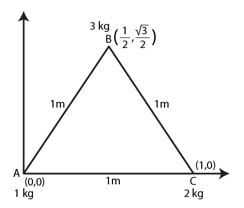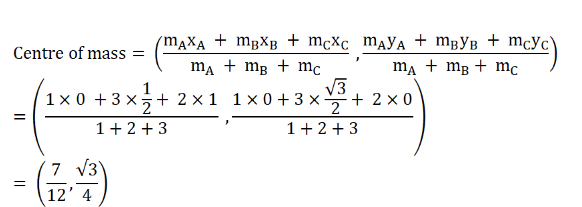This is the centre of mass from the point A.

Question 2: The structure of a water molecule is shown in figure (below). Find the distance of the center of mass of the molecule from the center of the oxygen atom.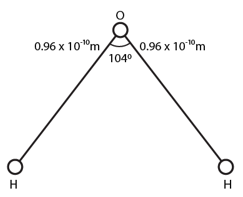Solution: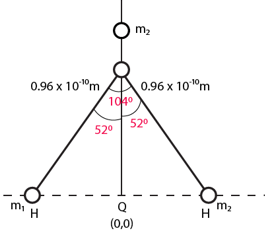Let θ be the origin of the system

From the figure:

m1 = 1 gm, m2 = 1 gm

x1 = -(0.96 x 10-10) sin 52o, x2 = -(0.96 x 10-10) sin 52o and x3 = 0

y1 = 0, y2 = 0 and y3 = (0.96 x 10-10) cos 52o

The position of the mass is: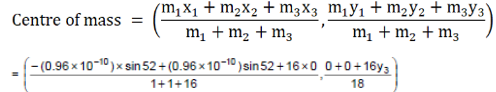= (0, (8/9) x 0.96 x 10-10 cos 52o )

Question 3: Seven homogeneous bricks, each of length L, are arranged as shown in figure (below). Each brick is displaced with respect to the one in contact by L/10. Find the x-coordinate of the center of mass relative to the origin shown.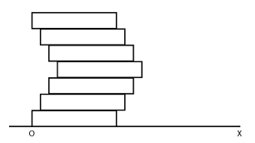Solution:

Let the mass of each brick be ‘m’. Centre of mass of each brick will be at its length L/2 . (Given)

Let O be the origin, the x coordinate of center of mass of the system: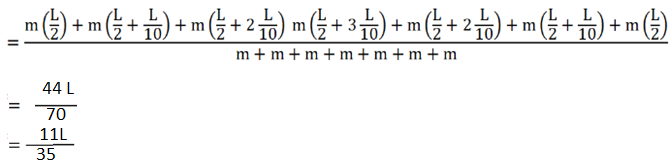Question 4: A uniform disc of radius R is put over another uniform disc of radius 2R of the same thickness and density. The peripheries of the two discs’ touch each other. Locate the center of mass of the system.

Solution: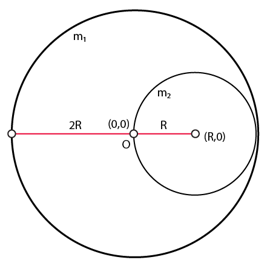Let the center of the bigger disc be the origin.

And density (mass/volume) of the both the discs be ‘ρ’ and thickness ‘t’.

Mass of the bigger disc = m1 = π (2R)2 t ρ

And mass of the smaller disc = m2 = π R2 t ρ

Position of center of mass of the bigger disc (x1, y1) = (0,0)

Position of center of mass of the smaller disc (x2, y2) = (R,0)

The position of the center of mass (x, y)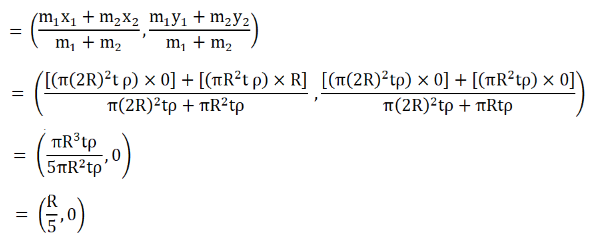Question 5: A disc of radius R is cut out from a larger disc of radius 2R in such a way that the edge of the hole touches the edge of the disc. Locate the center of mass of the residual disc.

Solution:

Let the center of the bigger disc be the origin.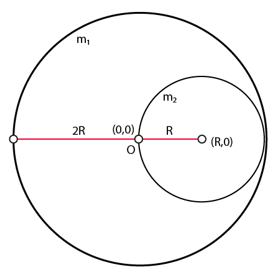Let the density of the both the discs be ‘ρ’ and thickness ‘t’.

Mass of the bigger disc = m1 = π (2R)2 t ρ

Mass of the maller disc = m2 = π R2 t ρ

Position of center of mass of the bigger disc (x1, y1) = (0,0)

Position of center of mass of the smaller disc (x2, y2) = (R,0)

Now, position of the new center of mass (x, y)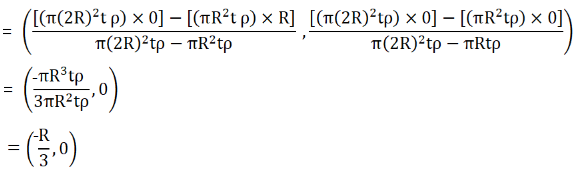Question 6: A square plate of edge d and a circular disc of diameter d are placed touching each other at the midpoint of an edge of the plate as shown in figure (below). Locate the center of mass of the combination, assuming same mass per unit area for the two plates.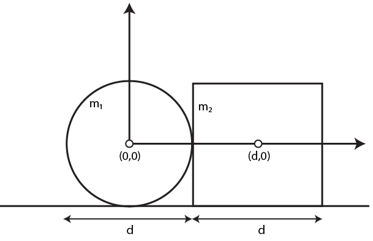Solution:

Given: Mass per unit area for the two plates is same.

Mass of the circular disc = m1 = mπ (d/2)2

Mass of the square plate= m2 = md2

Let origin to be at centre of the circular disc.

Position of the centre of mass :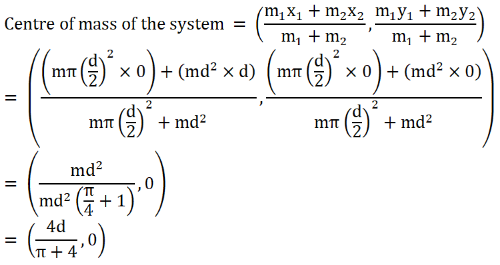The new centre of mass (4d/(π+4 ) right of the centre of circular disc.

Question 7: Calculate the velocity of the centre of mass of the system of particles shown in figure (below).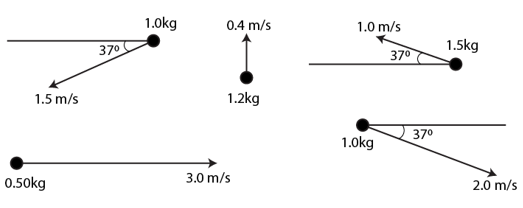Solution:

Velocity of centre of mass of the given system, v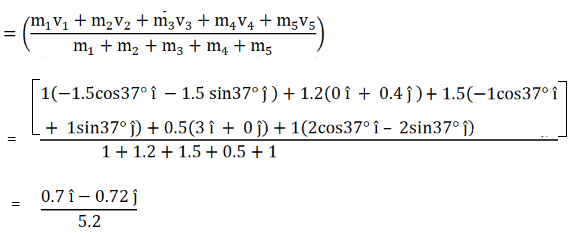Question 8: Two blocks of masses 10 kg and 20 kg are placed on the X-axis. The first mass is moved on the axis by a distance of 2 cm. By what distance should the second mass be moved to keep the position of the centre of mass unchanged?

Solution:

Let two masses are placed on the x-axis.

Centre of mass of the system be its origin,

Initial position of 10 kg mass = (-x1, 0)

Initial position of 20 kg mass = (x2, 0)

Final position of 10kg mass = (-x1+2, 0)

Final position of 20kg mass = (x2+x, 0)

Now,

x-cordinate of centre of mass with respect to initial position = (-10x1 + 20x2)/(10+20) = 0

x-cordinate of centre of mass with respect to final position = [10(-x1 +2) + 20(x2+x)]/(10+20) = 0

Equating above expressions, we have

(20+20x)/30 = 0

=> x = -1

The 2nd mass must be moved 1 cm towards the centre of mass to keep the centre of mass of the system unchanged.

Question 9: Two blocks of masses 10 kg and 30 kg are placed along a vertical line. The first block is raised through a height of 7 cm. By what distance should the second mass be moved to raise the centre of mass by 1 cm?

Solution:

Shift y-coordinate of the 10kg block = 7 cm

Shift in y-coordinate of the 20kg block = y cm

Shift in y-coordinate of centre of mass of the system = 1 cm

=> (10×7+30y)/(10=30) = 1

=> y = -1

The 30kg block should be displayed 1 cm downwards to raise the centre of mass through 1cm.

Question 10: Consider a gravity-free hall in which a tray of mass M, carrying a cubical block of ice of mass m and edge L, is at rest in the middle (figure below). If the ice melts, by what distance does the centre of mass of “the tray plus the ice” system descend?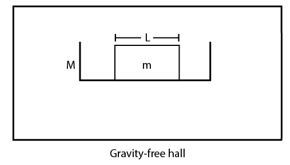Solution:

As hall is gravity free and the block of ice is at rest. (Given)

=> No external forces are acting on the block.

Therefore, the centre of mass of the system will stay the same.

So, the centre of mass of the system would not move.

Question 11: Find the centre of mass of a uniform plate having semicircular inner and outer boundaries of radii R1 and R2 (figure below).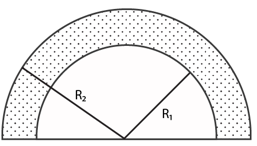Solution:

Let the mass per unit of the plate be m.

Mass of the semi-circular plate with radius R2 = m2 = π(R2)2 m

Mass of the semi-circular plate having radius R1 = m1 = π (R1)2 m

Consider, The x coordinate of centre of mass with zero.

y-coordinate of centre of mass of semi-circular plate having radius R2 = y2 = 4R2/3 π

y-coordinate of centre of mass of semi-circular plate having radius R1 = y1 = 4R1/3 π

The centre of mass of the plate will be on the symmetrical axis is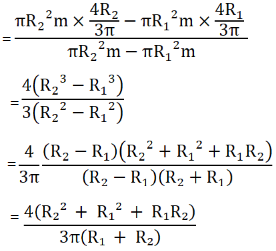Question 12: Mr. Verma (50 kg) and Mr. Mathur (60 kg) are sitting at the two extremes of a 4 m long boat (40 kg) Standing still in water. To discuss a mechanics problem, they come to the middle of the boat. Neglecting friction with water, how far does the boat move on the water during the process?

Solution:

Mass of Mr. Mathur = m1 = 60 kg

Mass of Mr. Verma = m2 = 50 kg

Mass of the boat = m3 = 40 kg

Taking the origin of the 1-D coordinate system to be at one extreme end of the boat where Mr. Verma and Mr. Mathur are sitting.

The centre of mass will be at a distance: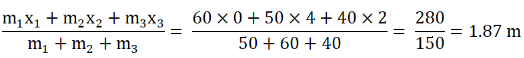Final centre of mass of the system when both of them are sitting at the centre of the boat = 2 m

Change in position of centre of mass of the boat = 2 – 1.87 = 0.13 m = 13 cm

Therefore, boat will move 13cm towards the side Mr. Verma was sitting.

Question 13: A cart of mass M is at rest on a frictionless horizontal surface and a pendulum bob of mass m hangs from the roof of the cart (figure below). The string breaks, the bob falls on the floor, makes several collisions on the fly and finally lands up in a small slot made in the floor. The horizontal distance between the string and the slot is L. Find the displacement of the cart during this process.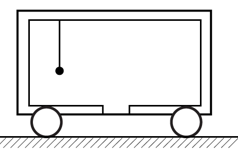Solution: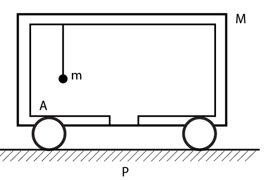Let the bob fall at A.

Let the mass of the bob = m

And mass of the cart = M

Initially, position of centre of mass of the system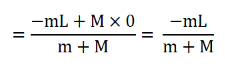Finally, position of centre of mass of the system = 0

Shift in centre of mass of the system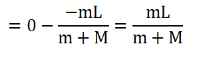But there is no external force in horizontal direction. So the cart displaces a distance mL/(m+M) towards right.

Question 14: The balloon, the light rope and the monkey shown in figure (below) are at rest in the air. If the monkey reaches the top of the rope, by what distance does the balloon descend? Mass of the balloon = M, mass of the monkey = m and the length of the rope ascended by the monkey = L.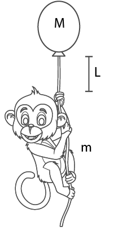Solution:

Initially, the balloon and monkey are at rest

And position of centre of mass of the system = -mL/m+M

Finally, when the monkey reaches the top, position of centre of mass of the system = 0

Shift in centre of mass of the system = mL/m+M

=> The balloon descends by a distance of mL/m+M

Question 15: Find the ratio of the linear momenta of two particles of masses 1.0 kg and 4.0 kg if their kinetic energies are equal.

Solution:

Let m1 and m2 masses of the particles and v1 and v2 are the velocities of the two particles.

Here m1 = 1 kg and m2 = 4 kg

Equating the kinetic energies of the particles, we have

(1/2) m1 v12 = (1/2) m2 v22

On substituting the values of m1 and m2

=> (v1/v2)2 = 4/1

Or (v1/v2) = 2/1

Thus, Ratio of their linear momenta = 1:2

Question 16: A uranium-238 nucleus, initially at rest, emits an alpha particle with a speed of 1.4 × 107 m/s. Calculate the recoil speed of the residual nucleus thorium-234. Assume that the mass of a nucleus is proportional to the mass number.

Solution:

Mass of the uranium-238 nucleus, m = 238 (Given)

Initial speed of the nucleus = u = 0 m/s

Final speed of the nucleus = v

Final speed of the alpha particle = v2 = 1.4 × 107 m/s

Mass of the nucleus after emission, m1 = 234

Mass of the alpha particle, m2 = 4

Applying conservation of momentum , mu = m1v1 + m2v2

238×0 = 234 v + 4 × 1.4 × 107

v = –2.39 × 105 m/s or –2.4 × 105 m/s (approx.)

Question 17: A man of mass 50 kg starts moving on the earth and acquires a speed of 1.8 m/s. With what speed does the earth recoil? Mass of earth = 6 × 1024 kg.

Solution:

Let m1 and m2 be the mass of man and mass of earth respectively.

m1 = 50 kg

m2 = 6×1024 kg

Let v1 and v2 be the speed of man and speed of earth

v1 = 1.8 m/s

By conservation of momentum, m1v1 = m2v2

50 × 1.8 = 6×1024 × v2

or v2 = 1.5 × 10-23 m/s

Question 18: A neutron initially at rest, decays into a proton, all electron and an antineutrino. The ejected electron has a momentum of 1.4 × 10-26 kg-m/s and the antineutrino 6.4 × 10-27 kg-m/s. Find the recoil speed of the proton (a) if the electron and the antineutrino are ejected along the same direction and (b) if they are ejected along perpendicular directions. Mass of the proton = 1.67 × X 10-27 kg.

Solution:

Given: Mass of the proton = 1.67 × X 10-27 kg.

Momentum of electron = 1.4 × 10-26 kg-m/s

Momentum of antineutrino = 6.4 × 10-27 kg-m/s

Let the velocity of proton be ‘v’ m/s

(a) Applying conservation of momentum,

Initial momentum of neutron = momentum of electron + momentum of proton + momentum of antineutrino

0 = 1.67 × 10-27 × v + 1.4 × 10-27 + 6.4 × 10-27

=> –1.67 × 10-27 × v = 20.4 × 10-27

or v = –12.2 m/s

Which is velocity of proton in direction opposite to electron and antineutrino.

(b) Resultant momentum of electron and antineutrino = √(142 + 6.42) x 10-27

= 15.4 x 10-27 kg m/s

Again,

Initial momentum of neutron = momentum of electron + momentum of proton + momentum of antineutrino

0 = 1.67 × 10-27 × v + 1.4 × 10-27 + 6.4 × 10-27

or

v = -9.2 m/s

Question 19: A man of mass M having a bag of mass m slips from the roof of a tall building of height H and starts falling vertically (figure below). When at a height h from the ground, he notices that the ground below him is pretty hard, but there is a pond at a horizontal distance x from the line of fall. In order to save himself he throws the bag horizontally (with respect to himself) in the direction opposite to the pond. Calculate the minimum horizontal velocity imparted to the bag so that the man lands in the water. If the man just succeeds to avoid the hard ground, where will the bag land?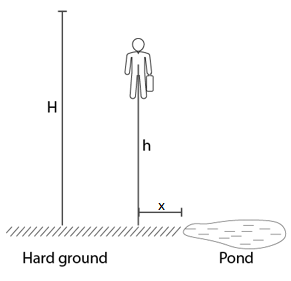Solution:

Mass of man = M and initial velocity = 0 and mass of bad = m

Let t be the total time man will take to reach the height h = time taken in falling through height H – time taken in falling through height (H-h)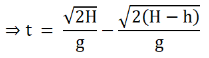Horizontal distance covered in this time = x

Horizontal velocity of the man, v2 = x/t

Now, mv1 = mv2

=>v1 = Mv2/m

=> Distance the bag travels = v1t = Mx/m

and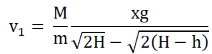Which is the minimum horizontal velocity imparted to the bag.

Question 20: A ball of mass 50 g moving at a speed of 2.0 m/s strikes a plane surface at an angle of incidence 45o. The ball is reflected by the plane at equal angle of reflection with the same speed. Calculate (a) the magnitude of the change in momentum of the ball (b) the change in the magnitude of the momentum of the ball.

Solution:

Mass of the ball, m = 50 g or 0.05 kg

Initial velocity of the ball = u = -2 cos45° î + 2 sin45° ĵ

Final velocity of ball after reflection = v = 2 cos45° î + 2 sin45° ĵ

(a) Change in momentum = m(v-u)

= 0.05×[(2cos45° î + 2sin45° ĵ ) – (-2cos45° î + 2sin45° ĵ)]

= 0.14 î

Magnitude of change in momentum = 0.2/√2 = 0.14 kg m/s

(b) Magnitude of initial momentum = m|u|

= 0.05×2

= 0.10 kg m/s

Magnitude of final momentum = m|v|

= 0.05 × 2

= 0.10 kg m/s

Change in magnitude of momentum = m|v| – m|u| = 0 kg m/s

Question 21: Light in certain cases may be considered as a stream of particles called photons. Each photon has a linear momentum h/λ where h is the Planck’s constant and λ is the wavelength of the light. A beam of light of wavelength λ is incident on a plane mirror at an angle of incidence θ. Calculate the change in the linear momentum of a photon as the beam is reflected by the mirror.

Solution:

The angle of reflection will be the same as angle of incidence, i.e. θ

Linear momentum of incident light = Pi= -(h/λ)cosθ î + (h/λ)sinθ ĵ

Linear momentum of reflected light = Pr= (h/λ)cosθ î + (h/λ)sinθ ĵ

Change in linear momentum = Pr – Pi

= [(h/λ)cosθ î + (h/λ)sinθ ĵ] – [-(h/λ)cosθ î + (h/λ)sinθ ĵ]

= -2(h/λ)cosθ î + 0 ĵ

=> Change in linear momentum is in x-direction = (2hcosθ)/λ

Question 22: A block at rest explodes into three equal parts. Two parts start moving along X and Y axes respectively with equal speeds of 10 m/s. Find the initial velocity of the third part.

Solution:

As the block is exploded only due to its internal energy. So, the net external force during this process is zero.

Pi = 0 kg m/s

Let the mass of each part be m kg and velocity of the third part be vxî + vyĵ

Final momentum = Pf = 10m î + 10m ĵ + m(vx î +vy ĵ)

By conservation of momentum, we have

pi = pf

0 = 10m î + 10m ĵ + m(vx î + vy ĵ)

vx î + vy ĵ = -10 î -10 ĵ

or vx = -10 and vy = -10

Magnitude of velocity of third part = √((-10) 2+(-10)2 ) = 10 √2 m/s

Angle with the horizontal = tan-1 (-10/10) = 135° from positive x axis or 45° below the x axis.

Question 23: Two fat astronauts each of mass 120 kg are travelling in a closed spaceship moving at a speed of 15 km/s in the outer space far removed from all other material objects. The total mass of the spaceship and its contents including the astronauts is 660 kg. If the astronauts do slimming exercise and thereby reduce their masses to 90 kg each, with what velocity will the spaceship move?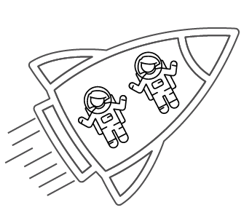Solution:

Two fat astronauts each of mass 120 kg are travelling in a closed spaceship moving at a speed of 15 km/s in the outer space far removed from all other material objects.

The spaceship is moving in vacuum and there is absolutely no external force acting on it.

So, the total mass of the spaceship remain unaffecting and also its velocity.

Velocity of the spaceship = same as before = 15 km/s

Question 24: During a heavy rain, hailstones of average size 1.0 cm in diameter fall with an average speed of 20 m/s. Suppose 2000 hailstones strike every square meter of a 10 m × 10 m roof perpendicularly in one second and assume that the hailstones

do not rebound. Calculate the average force exerted by the falling hailstones on the roof. Density of a hailstone is 900 kg/m3.

Solution:

Here d = 1 cm, v = 20 m/s and u = 0 and density = 900 kg/m3

Mass of a hailstone, m = Density×Volume

= 900 × 4/3 × π × (Radius)3

= 900×4/3× π×(0.5×10-2)3

= 0.471×10-3kg

Mass of 2000 hailstones, M = 2000×m = 0.942 kg

Rate of change in momentum per unit area = Rate of change of momentum

= M× (20 – 0)

= 18.84 N or 19 N (approx.)

Total force exerted on the roof = 19 × area of the roof

= 19 × 10 × 10 = 1900 N

Question 25: A ball of mass m is dropped onto a floor from a certain height. The collision is perfectly elastic and the ball rebounds to the same height and again falls. Find the average force exerted by the ball on the floor during a long time interval.

Solution:

Let a ball of mass m dropped onto a floor from a certain height “h”.

Velocity of the ball before the collision = √(2gh) and -√(2gh) after the collision.

Considering, v = √(2gh)

Time taken by the ball to hit the ground, t = v/g = √(2h/g)

Total time = 2t = 2 √(2h/g)

Average force exerted by the ball on the floor = Rate of change of momentum of the ball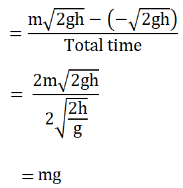Question 26: A railroad car of mass M is at rest on frictionless rails when a man of mass in starts moving on the car towards the engine. If the car recoils with a speed v backward on the rails, with what velocity is the man approaching the engine?

Solution:

Mass of the car = M and Mass of man = m

Initial velocity of the car, u2 = 0 and Initial velocity of man = u1

Velocity of man in the car = Recoil velocity of the car = v

Now,

mu1 + Mu2 = (m+M)v

[conservation of momentum]

mu1 + 0 = (m+M)v

u1 = (m+M)v/m

u1 = v (1 + M/m); is the velocity with which man approaches the engine.

Question 27: A gun is mounted on a railroad car. The mass of the car, the gun, the shells and the operator is 50 m where m is the mass of one shell. If the velocity of the shell with respect to the gun (in its state before firing) is 200 m/s, what is the recoil speed of the car after the second shot? Neglect friction.

Solution:

By conservation of momentum,

=> 0 = 49m×v + m×200

=> v = –200/49

After first shot, velocity of the car = (-200/49 ) m/s

After another shot, velocity of the car = (-200/48) m/s

Total recoiling velocity of the car = [-200(1/49 + 1/48)] m/s

Question 28: Two persons each of mass m are standing at the two extremes of a railroad car of mass M resting on a smooth track (figure below). The person on left jumps to the left with a horizontal speed u with respect to the state of the car before the jump. Thereafter, the other person jumps to the right, again with the same horizontal speed u with respect to the state of the car before his jump. Find the velocity of the car after both the persons have jumped off.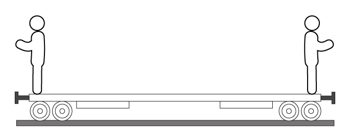Solution:

Let v be the recoil velocity of the railroad car when the man on the right jumps to the left.

Applying conservation of momentum,

⇒ 0 = –mu + (m+M)v

=> v = mu/(m+M)

Let v’ be the recoil velocity of the railroad car when the man on the left jumps to the right

=> 0 = mu – Mv’

=> v’ = mu/M

Now,

Net recoil velocity the car = v+v’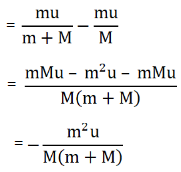Which is the velocity of the car after both people have jumped toward left.

Question 29: Figure (below) shows a small block of mass m which is started with a speed v on the horizontal part of the bigger block of mass M placed on a horizontal floor. The curved part of the surface shown is semicircular. All the surfaces are frictionless. Find the speed of the bigger block when the smaller block reaches the point A of the surface.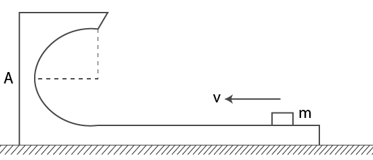Solution:

Using conservation of momentum =>mv + M×0 = (m+M)V

Where V be the speed of the bigger block.

mv = (m+M)V

=> V = mv/(m+M), which is speed of the bigger box.

Question 30: In a typical Indian Bugghi (a luxury cart drawn by horses), a wooden plate is fixed on the rear on which one person can sit. A bugghi of mass 200 kg is moving at a speed of 10 km/h. As it overtakes a school boy walking at a speed of 4 km/h, the boy sits on the wooden plate. If the mass of the boy is 25 kg, what will be the new velocity of the bugghi?

Solution:

Initial momentum of the school boy before sitting = 4×25 = 100 kg km/h

Initial momentum of the bugghi before the boy sat on it = 10×200 = 2000 kg km/h

Total initial momentum of the bugghi and the school boy = 2000+100 = 2100 kg km/h

Final momentum of the bugghi after the school boy sat on it = v×(100+25) = 125v kg km/h

Where v km/h be the new velocity of the bugghi.

Now, total linear momentum,

=> 125v = 2100

or v = (28/3) km/h

Question 31: A ball of mass 0.50 kg moving at a speed of 5.0 m/s collides with another ball of mass 1.0 kg. After the collision the balls stick together and remain motionless. What was the velocity of the 1.0 kg block before the collision?

Solution:

Initial momentum of the ball before collision = 0.50×5 = 2.5 kg m/s

Initial momentum of the other ball before collision = 1.0×v = v kg m/s

Where, v be the velocity of the other ball before collision.

Total initial momentum of the two balls = (2.5+v) kg m/s and both the balls come to rest after collision.

Final momentum of the two balls after collision = 0 kg m/s

By conservation of total linear momentum

=> 2.5 + v = 0 or v = –2.5 m/s

Question 32: A 60 kg man skating with a speed of 10 m/s collides with a 40 kg skater at rest and they cling to each other. Find the loss of kinetic energy during the collision.

Solution:

Total linear momentum of both the skaters before the collision = 60×10 + 40× 0 = 600 kg m/s

Total linear momentum of both skaters after the collision = v×(60+40) = 100v kg m/s

Where v be the velocity of both the skaters after they cling to each other.

Find v:

using conservation of total linear momentum,

100v = 600 or v = 6 m/s

Now,

Total KE of both skaters before the collision = (1/2) (60 x 102) + (1/2)(40-0) = 3000 Joule

And, Total KE of both skaters after the collision = (1/2) (60 x 40)(6)2 = 1800 Joule

Therefore, Total loss in kinetic energy during this collision = 3000 – 1800 = 1200 J

Question 33: Consider a head-on collision between two particles of masses m1 and m2. The initial speeds of the particles are u1 and u2 in the same direction. The collision starts at t = 0 and the particles interact for a time interval ∆t. During the collision, the speed of the first particle vanes as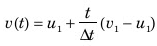Find the speed of the second particle as a function of time during the collision.

Solution:

The collision starts at t = 0 and the particles interact for a time interval ∆t.

During the collision the speed of the two particles of masses m1 and m2 are u(t) and u’ respectively.

By law of conservation of momentum

m1u1 + m2u2 = m1u(t)+ m2u’

since,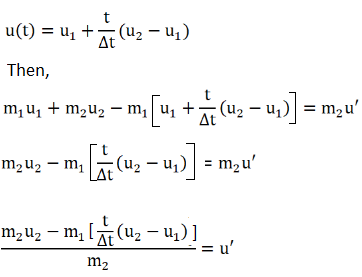Which is the speed of the second particle as a function of time during the collision.

Question 34: A bullet of mass m moving at a speed u hits a ball of mass M kept at rest. A small part having mass m’ breaks from the ball and sticks to the bullet. The remaining ball is found to move at a speed u1 in the direction of the bullet. Find the velocity of the bullet after the collision.

Solution:

From law of conservation of momentum

mv + 0 = (m’ + m)u’ + (M – m’)u1

or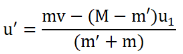Where u’ is the velocity of the bullet with frictional mass.

Question 35: A ball of mass m moving at a speed u makes a head-on collision with an identical ball at rest. The kinetic energy of the balls after the collision is three fourths of the original. Find the coefficient of restitution.

Solution:

Here mv1 + mv2 = mv + 0

Where v1 and v2 are the final velocities of the first ball and second ball and m is the mass of the second ball and v is the velocity of the first ball.

v1 + v2 = v and v1 – v2 = ev

Final KE = (3/4)(initial KE)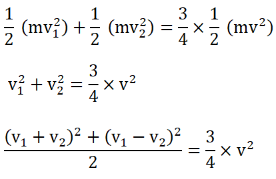Equations v1 + v2 = v and v1 – v2 = ev implies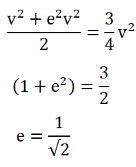Which is the coefficient of restitution.

Question 36: A block of mass 2.0 kg moving at 2.0 m/s collides head on with another block of equal mass kept at rest. (a) Find the maximum possible loss in kinetic energy due to the collision. (b) If the actual loss in kinetic energy is half of this maximum, find the coefficient of restitution.

Solution:

From law of conservation of momentum

mu + m’ x 0 = (m’ + m)v …(1)

Where m = mass of 1st block = 2kg, u = Initial speed before collision = 2 m/s and m’ = ass of the second block = 2kg

on putting values, we have

(1)=> v = 1 m/s

(a) Loss in kinetic energy in elastic collision = (1/2) mu2 + 0 – (1/2)(m’ + m)v2

= 2 J

(b) Actual loss = (1/2) x (maximum loss) = 2/2 = 1 J

Let v1 and v2 are the final velocities of the blocks of masses 2kg

Where v1 + v2 = u and v1 – v2 = eu.

Find Actual loss: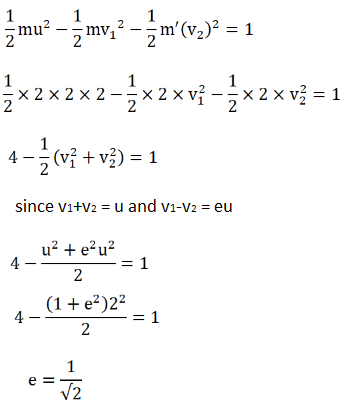Which is the coefficient of restitution.

Question 37: A particle of mass 100 g moving at an initial speed u collides with another particle of same mass kept initially at rest. If the total kinetic energy becomes 0.2 J after the collision, what could be the minimum and the maximum value of u.

Solution:

Here u is the initial speed and KE after collision is 0.2J

Now, Initial kinetic energy = (1/2) mu2

= (1/2) x 0.1 x u2

= 0.05 u2

We know, mu = mv1 = mv2

Where v1 and v2 are the final speed of first block and final speed of second block respectively.

v1 + v2 = u…..(1)

Again,

(v1 – v2) + L (a1-u2) =0

As u1 = u and u2 = 0

L a1 = (v2 -v1) …….(2)

Adding (1) and (2), we get

v1 = [u(1-L)/2]

and v2 = [u(1+L)/2]

Total kinetic energy after collision = (1/2)mv12 + (1/2) mv v22

=> 0.2 = v12 + v22 = 4

on substituting the values of v1 and v2, we get

u2 = 8/(1+L2)

For minimum value of u denominator should be maximum:

L=1 and u2 = 4 or u = 2m/s

For maximum value of u denominator should be minimum

L = 0 and u2 = 8 or u = 2√2 m/s

Question 38: Two friends A and B (each weighing 40 kg) are sitting on a frictionless platform some distance d apart. A rolls a ball of mass 4 kg on the platform towards B which B catches. Then B rolls the ball towards A and A catches it. The ball keeps on moving back and forth between A and B. The ball has a fixed speed of 5 m/s on the platform. (a) Find the speed of A after he rolls the ball for the first time, (b) Find the speed of A after he catches the ball for the first time. (c) Find the speeds of A and B after the ball has made 5 round trips and is held by A. (d) how many times can A roll the ball? (e) Where is the center of mass of the system “A + B + ball” at the end of the nth trip?

Solution: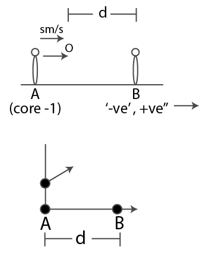Two friends A & B (each weighing 40kg) are sitting on a frictionless platform some distance d apart A rolls a ball of mass 4kg on the platform towards B, which B catches. Then B rolls the ball towards A and A catches it. The ball keeps on moving back & forth between A and B.

(a) Total momentum of man A and the ball will remain constant.

mu = (m+m)v1

0 = 20 – 40v

=> v = 0.5 m/s towards left

(b) When the man at B catches the ball, momentum between B and the ball will remain constant.

20 = 44v

=> v = (20/44) m/s

when B throws ball, then applying LCLM (Law of Conservation of Linear Momentum)

=> Mv1= -mu + Mv2

44 x (20/44) = -4 x 5 + 40 x v2

V2 = 1 m/s towards right

When A catches the ball, then applying LCLM

-mu + M(-v) = (M+m)v’

-4 x 5 + (-0.5) x 40 = -44 v’

v’= 10/11 m/s towards left

(c) speeds of A and B after the ball has made 5 round trips and is held by A

When A throws the ball

(M+m)v3 = mu – Mv4

44 x (10/11) = 4 x 5 – 40 x v4

v4 = 3/2 m/s towards left

Mv2 + mu = (M+m)v5

40 x 1 + 4 x 5 = 44 x v5

v5 = 15/11 m/s towards right

When B throws the ball,

(M+m)v5 = -mu + Mv6

44 x (66/44) = -4 x 5 + 40 x v6

V6 = 2 m/s towards right

When A catches the ball,

-mu – Mv4 = -(M+m)v’

-4 x 5 -40 x (3/2) = -44v’

v’= 20/11 m/s towards left

Similarly, After 5 round trips:

Velocity of A will be 50/11 and velocity of B will be 5 m/s

(d) After 6th round trip

Velocity of A = (60/11) m/s > 5 m/s, can’t reach the ball. So only can roll the ball six.

(e) Let the ball and body A at the initial position be at origin

= [Mv+mu+Md]/[M+M+m]

= [40×0 + 4×0+40xd]/[40+40+4]

= 10d/11

Question 39: A ball falls on the ground from a height of 2.0 m and rebounds up to a height of 1.5 m. Find the coefficient of restitution.

Solution:

we know, Velocity = u = √(2gh)

Where, g is the acceleration due to gravity and h is the height.

=> v = √(2×9.8×1.5)

Let u is the velocity of the ball when it approaches the ground

[Here g = 9.8 and h = 2]

=> u = √(2gh) = √(2×9.8×2)

Now, v + lu = 0

Where, l is the coefficient of restitution.

or l = -v/u

or l = √3/2

Question 40: In a gamma decay process, the internal energy of a nucleus of mass M decreases, a gamma photon of energy E and linear momentum E/c is emitted and the nucleus recoils. Find the decrease in internal energy.

Solution:

kinetic energy of nucleus = (1/2) mv2

Given that, internal energy of a nucleus of mass M decreases, and Linear momentum is E/c and the nucleus recoils.

=> KE = (1/2) m (E/mc) 2

KE = E/2mc2

Gamma photon energy is E.

Decrease in internal energy = E + E/2mc2

[As, decrease in internal energy will be equal to sum of energy limited by gamma photon and kinetic energy of nucleus]

Question 41: A block of mass 2.0 kg is moving on a frictionless horizontal surface with a velocity of 1.0 m/s (figure below) towards another block of equal mass kept at rest. The spring constant of the spring fixed at one end is 100 N/m. Find the maximum compression of the spring.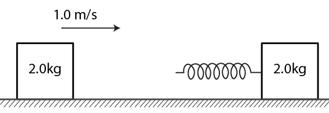Solution:

Let M1 and M2 masses of blocks which is 2 kg each.

Velocicty of 1st block = v1 = 1 m/s

Velocicty of 2nd block = v2 = 0 m/s

After collision velocity starts to decrease continuously and block 1 and block 2 starts moving together with common velocity.

using law of conservation energy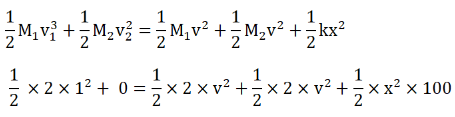=> 2v2 + 50 x2 = 1 ….(1)

When no external force in horizontal direction, the momentum should be conserved.

M1v1 + M2v2 = (M1 + M2)v

2x1 + 0 = 4xv

=> v = (1/2) m/s

(1)=> x = 10 cm, is the maximum compression of the spring.

Question 42: A bullet of mass 20 g travelling horizontally with a speed of 500 m/s passes through a wooden block of mass 10.0 kg initially at rest on a level surface. The bullet emerges with a speed of 100 m/s and the block slides 20 cm on the surface before

coming to rest. Find the friction coefficient between the block and the surface (figure below)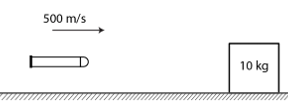Solution:

Here m = Mass of bullet = 20 g or 0.02 kg and M = Mass of block = 10 kg

Initial velocity of bullet = v1 = 500 m/s and Initial velocity of block = v2 = 0 m/s and Final velocity of bullet = v= 100 m/s

let v’ be the final velocity of block when bullet comes out

=> mv1 + Mv2 = mv + Mv’

=> 0.02 x 500 + 10 x0 = 0.02 x 100 + 10 x v’

=> v’ = 0.8 m/s

Now,

let μ friction coefficient between the block and the surface

Find μ:

As we are given, After moving a distance 0.2 it stops

0 – (1/2) x 10 x (0.8)2 = -μ x 10 x 10 x 0.2

[By work energy principle]

=> μ = 0.16

Question 43: A projectile is fired with a speed u at an angle θ above a horizontal field. The coefficient of restitution of collision between the projectile and the field is e. How far from the starting point, does the projectile makes its second collision with the field?

Solution: A projectile is fired with a speed u at an angle θ above a horizontal field. The coefficient of restitution of collision between the projectile and the field is e.

Let projection angle is Ө and projection velocity v.

g = 10 m/s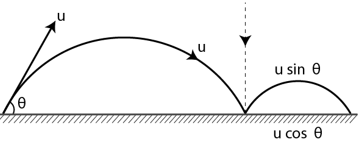e = (u sinθ)/v x v = eu sinθ

Velocity of projection for 2nd projectile motion and angle of projection,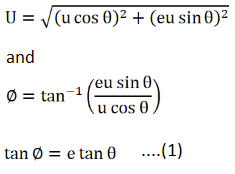Since equation for path of projectile motion

y = x tanφ – [gx2 sec2 φ]/2U2 ….(2)

Put y = 0, tan φ = e tanθ, sec2 φ = 1+ e2 tan2θ and

U = (u cos θ ) 2 + (eu sin θ ) 2

(2)=>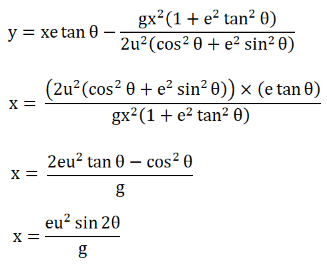Now,

From starting point O the projectile makes its second collision with the field at a distance x’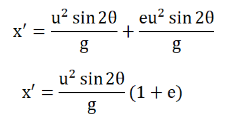Question 44: A ball falls on an inclined plane of inclination θ from a height h above the point of impact and makes a perfectly elastic Collision. Where will it hit the plane again?

Solution:

Let the striking velocity of the projectile with the inclined plane = v = √(2gh)

Now, the projectile makes an angle = α = (90o – 2θ)

velocity of the projectile = u = √(2gh)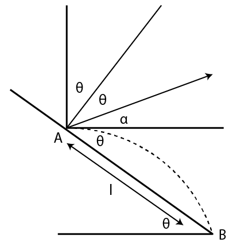Let AB = l

x = l cosθ and y = -l sinθ

using equation of trajectoryQuestion 45: Solve the previous problem if the coefficient of restitution is e. Use θ = 45°, e = 4/3 and h = 5 m.

Solution:

Let v be the striking velocity of the projectile with the inclined plane = v = √(2gh)

= √(2x10x5) = 10 m/s

After collision, let it make an angle θ with horizontal. Its horizontal component remain unchanged and velocity in perpendicular direction to the plane after collision is

vy = ev cosθ

= (3/4) x 10 x cos 45o

= (3.75)√2 m/s

and vx = v cos θ = (5/√2) m/s

So u = √(vx2 + vy2 )

= √(50+28.125)

= 8.83 m/s

Again, β be the angle of reflection from the wall.

=> β = tan-1 (3.75√2/5√2)

= tan-1(tan 37o) = 37o

Find angle of projection:

Let α be the angle of projection

α = 90 o – (45 o + 37 o) = 8 o

If l be the distance from it falls, coordinate are

x = l cosθ , y = -l sinθ

From equation of trajectory: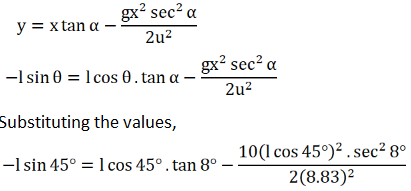Solving above, we have the value of l i.e.

l = 18.5 m

it will hit the plane again at 18.5 m along the incline.

Question 46: A block of mass 200 g is suspended through a vertical spring. The spring is stretched by 1.0 cm when the block is in equilibrium. A particle of mass 120 g is dropped on the block from a height of 45 cm. The particle sticks to the block after the impact. Find the maximum extension of the spring. Take g = 10 m/s2.

Solution:

The string is stretched by a distance x =1.00 cm= 0.01m.

In the equilibrium condition

Mg = kx

Where M = mass of block = 0.2 kg and g = 10 m/s

=> k = 200 N/m

The velocity with which the particle m will strike M is

u = √(2gh)

= √(2x10x0.45

= 3 m/s

After the collision the velocity of the particle and the block:

v = mu/(m+M)

=> v = (0.12×3)/0.32 = (9/8) m/s

From law of conservation of energy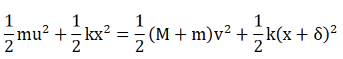Where, string be stretched through an extra deflection δ.

On substituting the values and solving, we have

δ = 6.1 cm

Question 47: A bullet of mass 25 g is fired horizontally into a ballistic pendulum of mass 5.0 kg and gets embedded in it (figure below). If the center of the pendulum rises by a distance of 10 cm, find the speed of the bullet.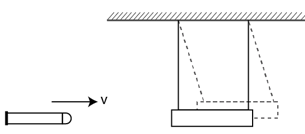Solution:

Let v be the final velocity and u is the strike pendulum.

Now, mu = (M+m)v

Here, m = 25 g = 0.025 kg, M = 5 kg

=> v = u/201

Using conservation of energy, we have

(1/2) (M+m)v2 = (M+m)gh

v2 = 2gh

Using value of v,

u = 280 m/s

Therefore, the bullet strikes pendulum with velocity u = 280 m/s.

Question 48: A bullet of mass 20 g moving horizontally at a speed of 300 m/s is fired into a wooden block of mass 500 g suspended by a long string. The bullet crosses the block and emerges on the other side. If the center of mass of the block rises through a height of 20.0 cm, find the speed of the bullet as it emerges from the block.

Solution:

Let the velocity of block is v’ and bullet emerges out with velocity is v.

Using law of conservation of momentum:

mu + Mu’ = mv + Mv’

or mu = Mv’ + mv …(1)

Work energy principle for the block after the collision

(1/2)Mu’2 – (1/2) Mv’2 = -Mgh

[here h = 0.2 m and u’ = 0 m/sec, M = 500gm = 0.5kg, and m = 20gm = 0.02kg and u = 300 m/sec.]

substituting the values and solving , we have

v’ = 2 m/s

(1)=> v = 250 m/s

Therefore, speed of the bullet as it emerges from the block is v= 250 m/s.

Question 49: Two masses m1 and m2 are connected by a spring of spring constant k and are placed on a frictionless horizontal surface. Initially the spring is stretched through a distance x0 when the system is released from rest. Find the distance moved by the two masses before they again come to rest.

Solution:

For the blocks to come to rest again, let the distance travelled by two blocks of mass m1 and m2 is x1 and x2 respectively.

As zero external force acts in horizontal direction,

m1x1 = m2x2 ….(1)

By law of conservation of energy

(1/2) kx2 = (1/2) k (x1 + x2 – x0)2

Thus, x0 = x1 + x2 – x0

or x1 = 2x0 – x2

(1)=> m1(2x0 – x2) = m2x2

x2 = 2m1x0/(m1 + m2)

similarly, we can find the value of x1

x1 = 2m2x0/(m1 + m2)

Question 50: Two blocks of masses m1 and m2 are connected by a spring of spring constant k (figure below). The block of mass m2 is given a sharp impulse so that it acquires a velocity u0 towards right. Find (a) the velocity of the center of mass, (b) the maximum elongation that the spring will suffer.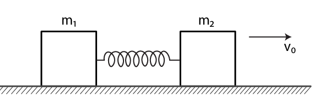Solution:

(a) Let vc be the velocity of center of mass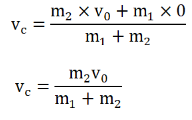(b)

when both the blocks will attain the velocity of centre of mass.

Let x be the maximum elongation of spring.

Change in kinetic energy = potential energy stored in spring